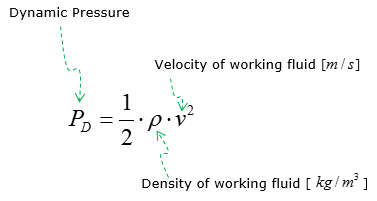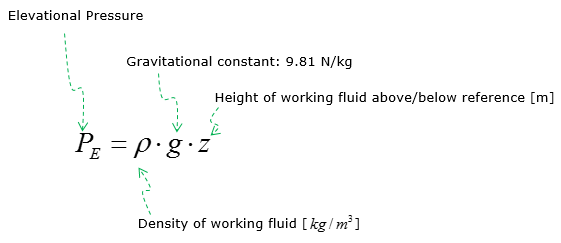Mechanical Engineering - Fluid Mechanics                                 Home : www.sharetechnote.com Fluid Mechanics : Pressure   The definition of pressure varies a little bit depending on the material and situations that we are dealing with. In many situation, the pressure is defined as  the force applied perpendicular to the surface of an object per unit area. It means that how much forces is applied to the unit area of an object.   In case of dealing with liquid, the pressure mean the compressive stress at some point within a fluid.  In this page, the term stress is mostly refer to 'Fluid Pressure'. It is mostly about liquid but it would apply to gases as well.   Static, Dynamic, and Elevational pressure of the working fluid make up Bernoulli’s equation  that represents conservation of energy of moving fluid per unit volume, also known as conservation of pressure as both energy per unit volume and pressure are measured in Pa   < Static Pressure >   Pressure experienced by the working fluid at rest or in motion when measurements are taken. Pressure gauge devices are used to measure static pressure in the working fluid.     < Dynamic Pressure >   Pressure due to the kinetic energy of the working fluid.< Elevational Pressure >   Pressure due to the potential energy of the working fluid.Reference :